# Integrals of Motion in T2 Coordinates

Motivated by the HNM82 derivation, in an accompanying chapter we have introduced a new T2 Coordinate System and have outlined a few of its properties. Here we explore whether an analytic prescription of the 3rd integral of motion can be formulated in a potential that conforms to this set of coordinates.

## Review

We begin by summarizing properties of the T2 Coordinate System that we have derived earlier. By defining the dimensionless angle,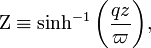$\Zeta \equiv \sinh^{-1}\biggl( \frac{qz}{\varpi} \biggr) ,$

the two key "T2" coordinates can be written as,

 χ1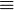$\equiv$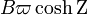$B \varpi \cosh\Zeta$ and χ2$\equiv$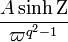$\frac{A \sinh\Zeta}{ \varpi^{q^2-1}}$ =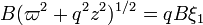$B ( \varpi^2 + q^2z^2 )^{1/2} = qB \xi_1$ =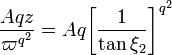$\frac{Aqz}{\varpi^{q^2}} = Aq \biggl[\frac{1}{\tan\xi_2} \biggr]^{q^2}$

Here are a variety of relevant partial derivatives: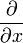$\frac{\partial}{\partial x}$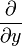$\frac{\partial}{\partial y}$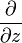$\frac{\partial}{\partial z}$ χ1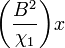$\biggl(\frac{B^2}{\chi_1}\biggr) x$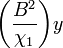$\biggl(\frac{B^2}{\chi_1}\biggr) y$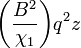$\biggl(\frac{B^2}{\chi_1}\biggr) q^2 z$ χ2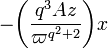$- \biggl( \frac{q^3 A z}{\varpi^{q^2+2}} \biggr) x$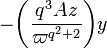$- \biggl( \frac{q^3 A z}{\varpi^{q^2+2}} \biggr) y$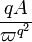$\frac{qA}{\varpi^{q^2}}$ χ3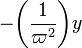$- \biggl( \frac{1}{\varpi^{2}} \biggr) y$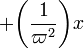$+ \biggl( \frac{1}{\varpi^{2}} \biggr) x$ 0

The scale factors are,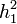$h_1^2$ =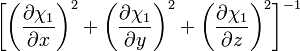$\biggl[ \biggl( \frac{\partial\chi_1}{\partial x} \biggr)^2 + \biggl( \frac{\partial\chi_1}{\partial y} \biggr)^2 + \biggl( \frac{\partial\chi_1}{\partial z} \biggr)^2 \biggr]^{-1}$ =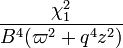$\frac{\chi_1^2}{B^4 (\varpi^2 + q^4 z^2)}$ =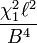$\frac{\chi_1^2 \ell^2}{B^4}$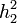$h_2^2$ =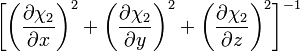$\biggl[ \biggl( \frac{\partial\chi_2}{\partial x} \biggr)^2 + \biggl( \frac{\partial\chi_2}{\partial y} \biggr)^2 + \biggl( \frac{\partial\chi_2}{\partial z} \biggr)^2 \biggr]^{-1}$ =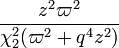$\frac{z^2 \varpi^2 }{\chi_2^2 (\varpi^2 + q^4 z^2)}$ =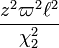$\frac{z^2 \varpi^2 \ell^2}{\chi_2^2}$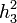$h_3^2$ =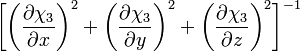$\biggl[ \biggl( \frac{\partial\chi_3}{\partial x} \biggr)^2 + \biggl( \frac{\partial\chi_3}{\partial y} \biggr)^2 + \biggl( \frac{\partial\chi_3}{\partial z} \biggr)^2 \biggr]^{-1}$ =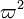$\varpi^2$ where,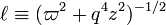$\ell \equiv (\varpi^2 + q^4 z^2)^{-1/2}$.

The position vector is,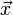$\vec{x}$ =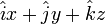$\hat{i}x + \hat{j}y + \hat{k}z$ =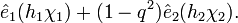$\hat{e}_1 (h_1 \chi_1) + (1 - q^2) \hat{e}_2 (h_2 \chi_2) .$

## Other Potentially Useful Differential Relations

In examining the equation of motion and searching for analytic representations of the 3rd integral of motion, we will need to know how each of the scale factors varies along each of the coordinate directions. Since our T2 coordinate system is an orthogonal system of coordinates, in general we can write,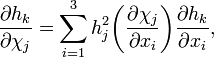$\frac{\partial h_k}{\partial\chi_j} = \sum_{i=1}^3 h_j^2 \biggl( \frac{\partial\chi_j}{\partial x_i} \biggr) \frac{\partial h_k}{\partial x_i} ,$

where xi are the three Cartesian coordinates. Analytic expressions for the first partial derivative in each term on the RHS,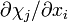$\partial\chi_j/\partial x_i$, can be obtained from the table shown above. To derive expressions for the second partial derivative in each RHS term, the following differential relations also will be useful:$\frac{\partial}{\partial x}$$\frac{\partial}{\partial y}$$\frac{\partial}{\partial z}$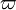$\varpi$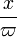$\frac{x}{\varpi}$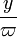$\frac{y}{\varpi}$ 0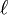$\ell$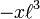$- x\ell^3$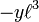$- y \ell^3$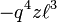$- q^4 z \ell^3$

Putting all of these expressions together, we derive the following: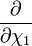$\frac{\partial}{\partial \chi_1}$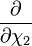$\frac{\partial}{\partial \chi_2}$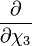$\frac{\partial}{\partial \chi_3}$ h1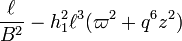$\frac{\ell}{B^2} - h_1^2 \ell^3 (\varpi^2 + q^6 z^2)$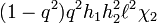$(1-q^2)q^2 h_1 h_2^2 \ell^2 \chi_2$ 0 h2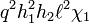$q^2 h_1^2 h_2 \ell^2 \chi_1$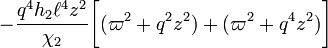$- \frac{q^4 h_2 \ell^4 z^2}{\chi_2} \biggl[ ( \varpi^2 + q^2 z^2 ) + ( \varpi^2 + q^4 z^2 ) \biggr]$ 0

## Time Derivatives

Assuming no mistakes have been made in our derivation of the above expressions, the time-derivative of the two key scale factors become,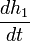$\frac{dh_1}{dt}$ =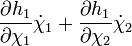$\frac{\partial h_1}{\partial\chi_1} \dot{\chi}_1 + \frac{\partial h_1}{\partial\chi_2} \dot{\chi}_2$ =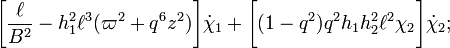$\biggl[ \frac{\ell}{B^2} - h_1^2 \ell^3 (\varpi^2 + q^6 z^2) \biggr]\dot{\chi}_1 + \biggl[ (1-q^2)q^2 h_1 h_2^2 \ell^2 \chi_2 \biggr]\dot{\chi}_2 ;$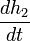$\frac{dh_2}{dt}$ =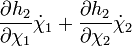$\frac{\partial h_2}{\partial\chi_1} \dot{\chi}_1 + \frac{\partial h_2}{\partial\chi_2} \dot{\chi}_2$ =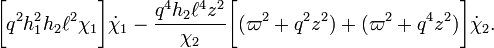$\biggl[ q^2 h_1^2 h_2 \ell^2 \chi_1 \biggr]\dot{\chi}_1 - \frac{q^4 h_2 \ell^4 z^2}{\chi_2} \biggl[ ( \varpi^2 + q^2 z^2 ) + ( \varpi^2 + q^4 z^2 ) \biggr]\dot{\chi}_2 .$

If the potential is only a function of the first T2 coordinate, χ1, then the 2nd component of the equation of motion states,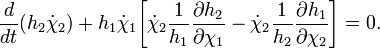$\frac{d}{dt}( h_2\dot{\chi}_2 ) + h_1 \dot{\chi}_1 \biggl[ \dot{\chi}_2 \frac{1}{h_1}\frac{\partial h_2}{\partial \chi_1} - \dot{\chi}_2 \frac{1}{h_2}\frac{\partial h_1}{\partial \chi_2} \biggr] = 0 .$

In other words,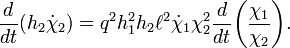$\frac{d}{dt}( h_2\dot{\chi}_2 ) = q^2 h_1^2 h_2 \ell^2 \dot{\chi}_1 \chi_2^2\frac{d}{dt}\biggl(\frac{\chi_1}{\chi_2}\biggr) .$

And the time derivative of a quantity that resembles the traditional angular momentum is,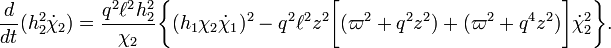$\frac{d}{dt}( h_2^2 \dot{\chi}_2 ) = \frac{q^2 \ell^2 h_2^2}{\chi_2} \biggl\{ (h_1 \chi_2 \dot{\chi}_1)^2 - q^2 \ell^2 z^2 \biggl[(\varpi^2 + q^2 z^2) + (\varpi^2 + q^4 z^2) \biggr]\dot{\chi}_2^2 \biggr\} .$

As has been discussed in an accompanying chapter, when q2 = 2 the product of χ1 and χ2 shows up as a key quantity when inverting the coordinate definitions. In particular, by defining,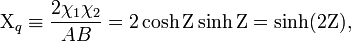$\Chi_q \equiv \frac{2\chi_1 \chi_2}{AB} = 2\cosh \Zeta \sinh\Zeta = \sinh(2\Zeta) ,$

and its companion,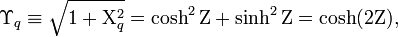$\Upsilon_q \equiv \sqrt{1 + \Chi_q^2} = \cosh^2\Zeta + \sinh^2\Zeta = \cosh(2\Zeta) ,$

we can write,$\varpi^2$ =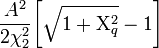$\frac{A^2}{2\chi_2^2} \biggl[ \sqrt{1 + \Chi_q^2} - 1 \biggr]$ =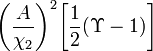$\biggl( \frac{A}{\chi_2} \biggr)^2 \biggl[\frac{1}{2} (\Upsilon - 1) \biggr]$ =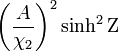$\biggl( \frac{A}{\chi_2} \biggr)^2 \sinh^2\Zeta$ =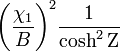$\biggl( \frac{\chi_1}{B} \biggr)^2 \frac{1}{\cosh^2\Zeta}$ z2 =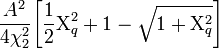$\frac{A^2}{4 \chi_2^2}\biggl[ \frac{1}{2} \Chi_q^2 +1 - \sqrt{1 + \Chi_q^2}\biggr]$ =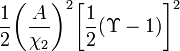$\frac{1}{2} \biggl( \frac{A}{\chi_2} \biggr)^2 \biggl[\frac{1}{2}(\Upsilon - 1)\biggr]^2$ =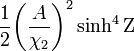$\frac{1}{2} \biggl( \frac{A}{\chi_2} \biggr)^2 \sinh^4\Zeta$ =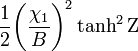$\frac{1}{2} \biggl( \frac{\chi_1}{B} \biggr)^2 \tanh^2\Zeta$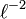$\ell^{-2}$ =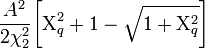$\frac{A^2}{2\chi_2^2} \biggl[ \Chi_q^2 + 1 - \sqrt{ 1 + \Chi_q^2} \biggr]$ =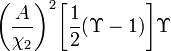$\biggl( \frac{A}{\chi_2} \biggr)^2 \biggl[ \frac{1}{2} (\Upsilon - 1)\biggr] \Upsilon$ =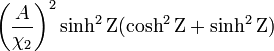$\biggl( \frac{A}{\chi_2} \biggr)^2 \sinh^2\Zeta ( \cosh^2\Zeta + \sinh^2\Zeta )$ =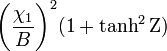$\biggl( \frac{\chi_1}{B} \biggr)^2( 1 + \tanh^2\Zeta )$

Hence, potentially useful expressions for the scale factors are,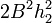$2B^2 h_2^2$ =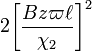$2 \biggl[\frac{B z \varpi \ell}{\chi_2} \biggr]^2$ =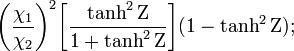$\biggl( \frac{\chi_1}{\chi_2} \biggr)^2 \biggl[ \frac{\tanh^2\Zeta}{1+\tanh^2\Zeta} \biggr](1-\tanh^2\Zeta) ;$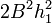$2B^2 h_1^2$ =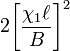$2 \biggl[\frac{\chi_1 \ell}{B} \biggr]^2$ =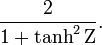$\frac{2}{1+\tanh^2\Zeta} .$

Notice that, because it is expressible entirely in terms of Χq, the variable Ζ is a function only of the product of the two key coordinates. Hence, the scale factor h1 is only a function of the product of the coordinates while h2 depends on the ratio, as well as the product of the two coordinates. In this context, note that Ζ can be derived from the product of the two key coordinates via one of the following two relations: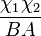$\frac{\chi_1\chi_2}{BA}$ =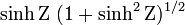$\sinh\Zeta ~(1 + \sinh^2\Zeta)^{1/2}$ =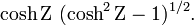$\cosh\Zeta ~(\cosh^2\Zeta - 1)^{1/2} .$